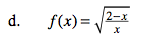### Home > CALC > Chapter Ch1 > Lesson 1.2.4 > Problem1-71

1-71.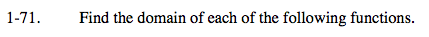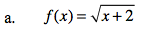$\text{If the domain of the parent graph, } y=\sqrt{x}, \text{ is } x \geq 0,\text{ what is the domain of }f(x)?$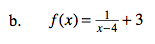$\text{If the domain of the parent graph, } y=\frac{1}{x}, \text{ is } x \neq 0,\text{ what is the domain of }f(x)?$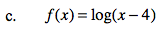$\text{If the domain of the parent graph, } y=\text{ log}{x}, \text{ is } x > 0,\text{ what is the domain of }f(x)?$